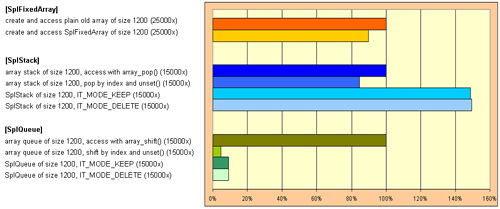# 本文由 开发之家 – wxz1425255448 发布，转载请注明出处，如有问题请联系我们！PHP标准库(PHP SPL)详解

SPL，PHP 标准库（Standard PHP Library） ，此从 PHP 5.0 起内置的组件和接口，并且从 PHP5.3 已逐渐的成熟。SPL 其实在所有的 PHP5 开发环境中被内置，同时无需任何设置。

SPL 对 PHP 引擎进行了扩展，例如 ArrayAccess、Countable 和 SeekableIterator 等接口，它们用于以数组形式操作对象。同时，你还可以使用 RecursiveIterator、ArrayObejcts 等其他迭代器进行数据的迭代操作。

SPL提供了一组标准数据结构：

• SplStack

• SplQueue

?

 123456789101112131415161718192021222324252627282930313233343536373839404142434445464748 `SplDoublyLinkedList ``implements` `Iterator , ArrayAccess , Countable { `` ``public` `__construct ( void )`` ``public` `void add ( mixed ``\$index` `, mixed ``\$newval` `)`` ``//双链表的头部节点`` ``public` `mixed top ( void )`` ``//双链表的尾部节点`` ``public` `mixed bottom ( void )`` ``//双联表元素的个数`` ``public` `int ``count` `( void )`` ``//检测双链表是否为空`` ``public` `bool isEmpty ( void )`` ``//当前节点索引`` ``public` `mixed key ( void )`` ``//移到上条记录`` ``public` `void prev ( void )`` ``//移到下条记录`` ``public` `void next ( void )`` ``//当前记录`` ``public` `mixed current ( void )`` ``//将指针指向迭代开始处`` ``public` `void ``rewind` `( void )`` ``//检查双链表是否还有节点`` ``public` `bool valid ( void )  `` ``//指定index处节点是否存在`` ``public` `bool offsetExists ( mixed ``\$index` `)`` ``//获取指定index处节点值`` ``public` `mixed offsetGet ( mixed ``\$index` `)`` ``//设置指定index处值`` ``public` `void offsetSet ( mixed ``\$index` `, mixed ``\$newval` `)`` ``//删除指定index处节点`` ``public` `void offsetUnset ( mixed ``\$index` `) `` ``//从双链表的尾部弹出元素`` ``public` `mixed pop ( void )`` ``//添加元素到双链表的尾部`` ``public` `void push ( mixed ``\$value` `)  `` ``//序列化存储`` ``public` `string serialize ( void )`` ``//反序列化`` ``public` `void unserialize ( string ``\$serialized` `)`` ``//设置迭代模式`` ``public` `void setIteratorMode ( int ``\$mode` `)`` ``//获取迭代模式SplDoublyLinkedList::IT_MODE_LIFO (Stack style) SplDoublyLinkedList::IT_MODE_FIFO (Queue style)`` ``public` `int getIteratorMode ( void )`` ``//双链表的头部移除元素`` ``public` `mixed shift ( void )`` ``//双链表的头部添加元素`` ``public` `void unshift ( mixed ``\$value` `)``}`

?

 12345678910111213141516171819202122232425262728293031323334353637383940414243444546474849505152535455565758596061626364 `\$list` `= ``new` `SplDoublyLinkedList();`` ``\$list``->push(``'a'``);`` ``\$list``->push(``'b'``);`` ``\$list``->push(``'c'``);`` ``\$list``->push(``'d'``); `` ``\$list``->unshift(``'top'``);`` ``\$list``->shift(); `` ``\$list``->``rewind``();``//rewind操作用于把节点指针指向Bottom所在的节点`` ``echo` `'curren node:'``.``\$list``->current().``"
"``;``//获取当前节点`` ``\$list``->next();``//指针指向下一个节点`` ``echo` `'next node:'``.``\$list``->current().``"
"``;`` ``\$list``->next();`` ``\$list``->next();`` ``\$list``->prev();``//指针指向上一个节点`` ``echo` `'next node:'``.``\$list``->current().``"
"``;`` ``if``(``\$list``->current())``   ``echo` `'current node is valid
'``;`` ``else``   ``echo` `'current node is invalid
'``;`` ``if``(``\$list``->valid())``//如果当前节点是有效节点，valid返回true``   ``echo` `"valid list
"``;`` ``else``   ``echo` `"invalid list
"``;`` ``var_dump(``array``(``   ``'pop'` `=> ``\$list``->pop(),``   ``'count'` `=> ``\$list``->``count``(),``   ``'isEmpty'` `=> ``\$list``->isEmpty(),``   ``'bottom'` `=> ``\$list``->bottom(),``   ``'top'` `=> ``\$list``->top()`` ``));`` ``\$list``->setIteratorMode(SplDoublyLinkedList::IT_MODE_FIFO);`` ``var_dump(``\$list``->getIteratorMode());`` ``for``(``\$list``->``rewind``(); ``\$list``->valid(); ``\$list``->next()) {``   ``echo` `\$list``->current().PHP_EOL;`` ``}`` ``var_dump(``\$a` `= ``\$list``->serialize());`` ``//print_r(\$list->unserialize(\$a));`` ``\$list``->offsetSet(0,``'new one'``);`` ``\$list``->offsetUnset(0);`` ``var_dump(``array``(``   ``'offsetExists'` `=> ``\$list``->offsetExists(4),``   ``'offsetGet'` `=> ``\$list``->offsetGet(0),`` ``));`` ``var_dump(``\$list``);`` ``//堆栈，先进后出`` ``\$stack` `= ``new` `SplStack();``//继承自SplDoublyLinkedList类`` ``\$stack``->push(``"a
"``);`` ``\$stack``->push(``"b
"``);`` ``echo` `\$stack``->pop();`` ``echo` `\$stack``->pop();`` ``echo` `\$stack``->offsetSet(0,``'B'``);``//堆栈的offset=0是Top所在的位置，offset=1是Top位置节点靠近bottom位置的相邻节点，以此类推`` ``\$stack``->``rewind``();``//双向链表的rewind和堆栈的rewind相反，堆栈的rewind使得当前指针指向Top所在的位置，而双向链表调用之后指向bottom所在位置`` ``echo` `'current:'``.``\$stack``->current().``'
'``;`` ``\$stack``->next();``//堆栈的next操作使指针指向靠近bottom位置的下一个节点，而双向链表是靠近top的下一个节点`` ``echo` `'current:'``.``\$stack``->current().``'
'``;`` ``echo` `'

'``;`` ``//队列，先进先出`` ``\$queue` `= ``new` `SplQueue();``//继承自SplDoublyLinkedList类`` ``\$queue``->enqueue(``"a
"``);``//插入一个节点到队列里面的Top位置`` ``\$queue``->enqueue(``"b
"``);`` ``\$queue``->offsetSet(0,``'A'``);``//堆栈的offset=0是Top所在的位置，offset=1是Top位置节点靠近bottom位置的相邻节点，以此类推`` ``echo` `\$queue``->dequeue();`` ``echo` `\$queue``->dequeue();`` ``echo` `"

"``;`

?

 12345678 ``

?

 1234567891011121314151617181920212223242526 `getInnerIterator();``    ``if` `(``\$item``->isFile() && ``        ``in_array(``pathinfo``(``\$item``->getFilename(), PATHINFO_EXTENSION), ``\$this``->ext)) {``      ``return` `TRUE;``    ``}``  ``}``}``// 实例化``foreach` `(``new` `RecursiveFileFilterIterator(``'/path/to/something'``) ``as` `\$item``) {``  ``echo` `\$item` `. PHP_EOL;``}``?>`

• RecursiveIterator

• RecursiveIteratorIterator

• OuterIterator

• IteratorIterator

• FilterIterator

• RecursiveFilterIterator

• ParentIterator

• SeekableIterator

• LimitIterator

• GlobIterator

• CachingIterator

• RecursiveCachingIterator

• NoRewindIterator

• AppendIterator

• RecursiveIteratorIterator

• InfiniteIterator

• RegexIterator

• RecursiveRegexIterator

• EmptyIterator

• RecursiveTreeIterator

• ArrayIterator

SplFixedArray

SPL 还内置了一系列的数组操作工具，例如可以使用 SplFixedArray 实例化一个固定长度的数组。那么为什么要使用它？因为它更快，甚至它关系着你的工资问题 :)## 评论（0条）请登录后评论# wxz1425255448

0

0

0

( 此人很懒并没有留下什么~~ )